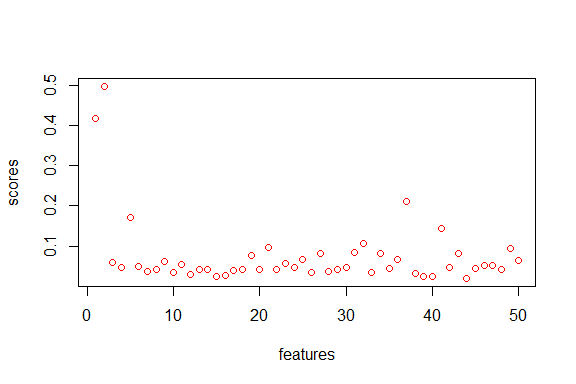# demo-fusionclust

This vignette provides a quick demo of the functionalities of the fusionclust package.

## Example 1 - track the big merges and estimate the number of clusters

This example demonstrates how to estimate the number of clusters using bmt and nclust.

# Generate bimodal data
set.seed(42)
x<- c(rnorm(1000,-2,1), rnorm(1000,2,1))

# Run Big Merge Tracker on x
library("fusionclust")
bmt_output<-bmt(x)
##  "BEWARE: there is a big merge when total no. of clusters are  51"

Now, estimate the number of clusters.

# Estimate the number of clusters - k
k<- nclust(bmt_output)
k
##  2

## Example 2 - rank and screen the p features in a clustering problem

This example demonstrates feature ranking and screening using cosci_is and cosci_is_select.

# Generate n by p=50 design matrix with 2 signals and 48 noise features
n<-1000
features<-1:50
set.seed(42)
noise<- matrix(rnorm(48000,0,1),nrow=1000,ncol=48)

# signal 1 ~ mixture of Normals
set.seed(42)
s1<- c(rnorm(n/2,-1.5,1),rnorm(n/2,1.5,1))

# signal 2 ~ mixture of Log Normal and Normal
set.seed(42)
s2<- c(rlnorm(n/2,0.2,0.35),rnorm(n/2,4,0.5))

# put it all together
x<- cbind(s1,s2,noise)

Now, conduct feature ranking using cosci_is.

library("fusionclust")
scores<- cosci_is(x,0)

# plot the scores and see which features have higher scores
plot(features,scores,type="p",col="red")Screen out the noise features using cosci_is_select.

features<-cosci_is_select(scores,0.9)
features$selected ##  1 2 5 37 41 You can also get an implicit threshold value. imp.thresh<- min(scores[features$selected])
imp.thresh
##  0.143